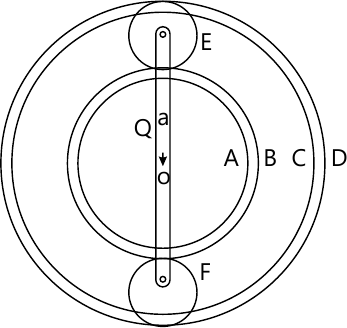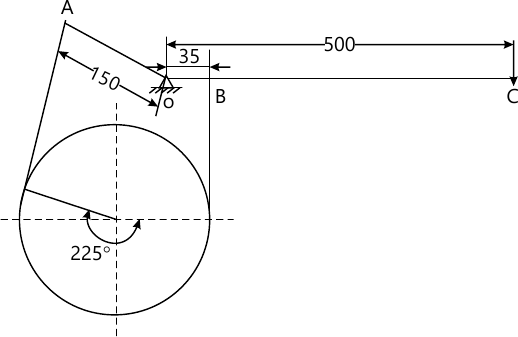MORE IN Theory of Machines - 2
MU Mechanical Engineering (Semester 5)
Theory of Machines - 2
December 2015
Total marks: --
Total time: --
INSTRUCTIONS
(1) Assume appropriate data and state your reasons
(2) Marks are given to the right of every question
(3) Draw neat diagrams wherever necessary

1 (a) What do you understand by dry clutch and wet clutch?
5 M
1 (b) Define dynamically equivalent system. State the condition is necessary to make two systems dynamically equivalent.
5 M
1 (c) Explain the necessity of gear box in automobile.
5 M
1 (d) Explain the following terms with reference to a governor
i) Sensitiveness ii) Stability iii) Isochronism iv) Coefficient of insensitiveness
5 M
1 (e) What do you understand by gyroscopic couple? Derive a formula for its magnitude.
5 M

2 (a) A cone clutch with a semi-cone angle of 15° transmits 10kW at 600rpm. The normal pressure intensity between the surfaces in contact is not to exceed 100 kN/m2. The width of the friction surfaces is half of mean diameter. Assume μ=0.25. Determine
(i) the outer and inner diameter of the plate.
(ii) Width of the cone face.
iii) the axial force to engage the clutch.
10 M
2 (b) In the epicyclic gear train show in Fig 1. The compound wheels A and B as well as internal wheels C and D rotate independently about the axis 0. The wheels E and F rotate on the pins fixed to the arm 'a'. All the wheels are of the same module. The number of teeth on the wheels are
TA=52, TB=56, TE=TF=36.
Determine the speed of C, if (i) the wheel D fixed and arm 'a' rotates at 200 rpm clockwise (ii) the wheel D rotates at 20 rpm counter-clockwise and arm 'a' rotates at 200 rpm clockwise.10 M

3 (a) A horizontal gas engine running at 210 rpm has a bore of 220 mm and a stroke of 440 mm. The connecting rod is 924 mm long and the reciprocating parts weigh 20kg. when the crank has turned through an angle of 30° from the inner dead center, the gas pressure on the cover and the crank sides are 500 kn/m2 and 60 kN/m2 respectively. Diameter of the piston is 40mm. Determine
(i) turning moment on the crank shaft.
(ii) thrust on the bearings.
acceleration of the wheel which has a mass of 8kg and radius of gyration of 600 mm while the power of engine is 22 kW.
8 M
3 (b) In a spring-loaded Hartnell type of governor, the mass of each ball is 4 kg and lift of the sleeve is 40 mm. The governor begins to float at 200 rpm when the radius of the ball path is 90 mm. The mean working speed of the governor is 16 times the range of speed when friction is neglected. The lengths of the ball and roller arms of the bell crank lever are 100 mm and 80 mm respectively. The pivot centre and axis of the governor are 115 mm apart. Determine the initial compression of the spring, taking into account the obliquity of arms.
Assuming the friction at the sleeve to be equivalent to a force of 15 N, determine the total alteration in speed before the sleeve begins to move from the mid-position.
12 M

4 (a) Each wheel of a motorcycle is of 600 mm diameter and has a moment of inertia of 1.2 kg-m2. The total mass of the motorcycle and the rider is 180 kg and combined centre of mass is 580 mm above the ground level when the motor cycle is upright. The moment of inertia of the rotating parts of the engine is 0.2 kg-m2. The engine speed is 5 times the speed of the wheels and is in the same sense. Determine the angle of heel necessary when the motor cycle takes a turn of 35m radius at a speed of 54km/h.
10 M
4 (b) Single plate clutch transmits 25 kW at 900 rpm. The maximum pressure intensity between the plates is 85 kN/m2. The outer diameter of the plate is 360 mm. Both the sides of the plate are effective and the coefficient of friction is 0.25. Determine (i) Inner diameter of the plate, and (ii) axial force to engage the clutch.
10 M

5 (a) A differential band brake, as shown in Fig. 2 has an angle of contact of 225°. The band has a compressed woven lining and bears against a cast iron drum of 350 mm diameter. The brake is to sustain a torque of 350 N-m and the coefficient of friction between the band and drum is 0.30. Find (a) The necessary force (F) for the clockwise and anticlockwise rotation of the drum; and (b) The value of 'OA' for the brake to be self-locking, when the drum rotates clockwise.12 M
5 (b) A cast iron flywheel is requires to absorb 25000 Nm of energy as speed is increased from 120 to 125 rpm. If the wheel is to be solid disc having a diameter 8 times its thickness. Determine its diameter. Density of C.I.=7200 kg/m3.
8 M

6 (a) A loaded porter governor has 4 links each 25 cm long. 2 revolving masses each weighing 30N and a central dead weight weighing 200N. All the links are attached to respective sleeves at radial distance of 4 cm from the axis of rotation. The masses revolve at a radius of 15 cm at minimum speed and at a radius of 20 cm at maximum speed. Determine the range of speed.
10 M
6 (b) The turbine rotor of ship has a mass of 900 kg and radius of gyration 600 mm. it rotates at 1800 rpm clockwise looking from the stern. Determine gyroscopic couple and its effect when; (i) the ship is travelling at 40 km/hr and steers to left in a curve of 100 m radius; (ii) the ship is pitching and the bow is descending with maximum velocity. The pitching is simple harmonic, the periodic time being 30 seconds and total angular moment between the extreme positions is 12°.
10 M

More question papers from Theory of Machines - 2